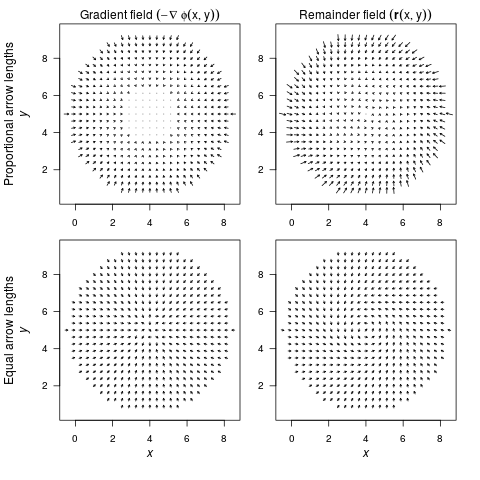In this vignette, we will extend example 2 from the R article and include the code to produce the figures.

### Example 2: A model with a limit cycle

``````    library(QPot)
var.eqn.x <- "-(y-beta) + mu*(x-alpha)*(1-(x-alpha)^2-(y-beta)^2) "
var.eqn.y <- "(x-alpha) + mu*(y-beta)*(1-(x-alpha)^2-(y-beta)^2)"
model.parms <- c(alpha = 4, beta = 5, mu = 0.2)
parms.eqn.x <- Model2String(var.eqn.x, parms = model.parms, supress.print = TRUE)
parms.eqn.y <- Model2String(var.eqn.y, parms = model.parms, supress.print = TRUE)
``````

### Step 1: Analyze the deterministic skeleton

To analyze our system of differential equations, we use the package phaseR, which requires the system of equations to be implemented as a function. Our function Model2String() was made to help users of QPot transition from their use of previous packages requiring this function format.

``````    require(phaseR)
model.ex2 <- function(t, y, parameters){
x <- y
y <- y
alpha <- parameters["alpha"]
beta <- parameters["beta"]
delta <- parameters["delta"]
kappa <- parameters["kappa"]
gamma <- parameters["gamma"]
mu <- parameters["mu"]
dx <- -(y-beta) + mu*(x-alpha)*(1-(x-alpha)^2-(y-beta)^2)
dy <- (x-alpha) + mu*(y-beta)*(1-(x-alpha)^2-(y-beta)^2)
list(c(dx,dy))
}
``````
``````    # draws the vector field
flowField(deriv = model.ex2, xlim = c(2, 7), ylim = c(2, 7), parameters = model.parms,
add = F, points = 30, col = "grey70", ann = F, arrow.head = 0.025, frac = 1.1,
xaxs = "i", yaxs = "i", las = 1)
# draw the nullclines and suppress the output by assigning it to a variable
supp.print <- nullclines(deriv = model.ex2, xlim = c(2, 7), ylim = c(2, 7),
parameters = model.parms, col = c("blue", "red"), points = 250)
# draw and label the equilibria
# open circles are unstable, black are stable
points(4,5 , pch = 21 , col = "black" , bg = "white" , cex = 1.5)
text(4,5 , labels = expression(italic(e)) , adj = c(0,-.25) , cex = 1.5)
traj <- trajectory(model.ex2,y0=c(runif(1,2,7),runif(1,2,7)) , tlim = c(2,7), xlim = c(2,7) , ylim = c(2,7) , parameters = model.parms , t.end = 250, lwd = 1.5 , pch = 16)
traj <- trajectory(model.ex2,y0=c(runif(1,2,7),runif(1,2,7)) , tlim = c(2,7), xlim = c(2,7) , ylim = c(2,7) , parameters = model.parms , t.end = 250, lwd = 1.5 , pch = 16)
traj <- trajectory(model.ex2,y0=c(runif(1,2,7),runif(1,2,7)) , tlim = c(2,7), xlim = c(2,7) , ylim = c(2,7) , parameters = model.parms , t.end = 250, lwd = 1.5 , pch = 16)
# label the x and y axis
mtext(expression(italic(x)), side = 1, line = 2.5)
mtext(expression(italic(y)), side = 2, line = 2.5)
``````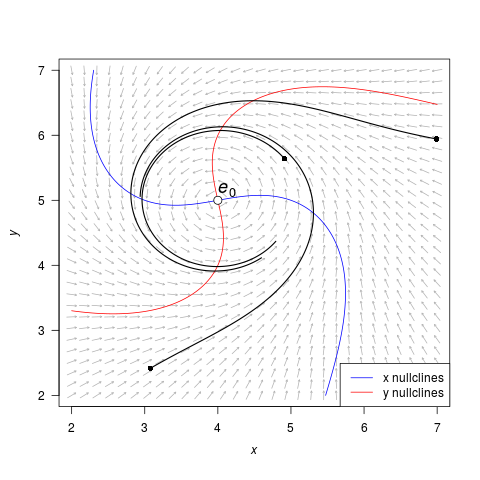### Step 2: Stochastic simulation

In order to simulate the stochastic equations, we need:

``````    model.state <- c(x = 3, y = 3)  # initial condition
model.sigma <- 0.1              # the level of noise
model.time <- 500       #2500       # the length of the simulation
model.deltat <- 0.005           # the time step
``````

Then we can simulate the equations using TSTraj():

``````    ts.ex2 <- TSTraj(y0 = model.state, time = model.time,
deltat = model.deltat, x.rhs = var.eqn.x, y.rhs = var.eqn.y,
parms = model.parms, sigma = model.sigma)
``````

The function TSPlot allows us to easily view the output from TSTraj(). The default plot is the time series plotted through time with a histogram:

``````    TSPlot(ts.ex2, deltat = model.deltat)
``````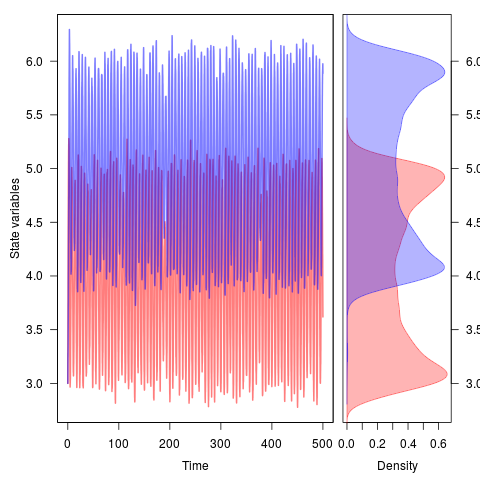We can view the times series plotted along on the state variables by setting dim = 2:

``````    TSPlot(ts.ex2, deltat = model.deltat, dim = 2)
``````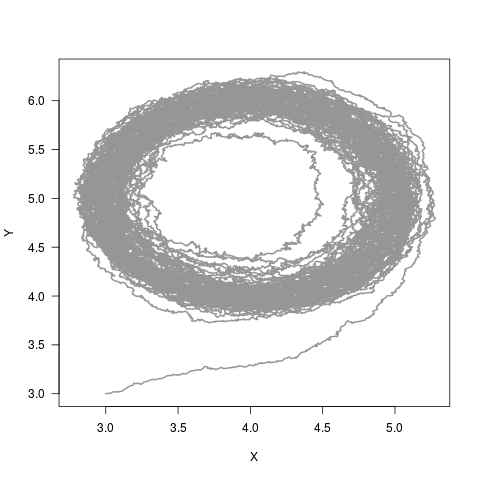If we only want to see the histograms, we can see them in one dimensional space

``````    TSDensity(ts.ex2, dim = 1)
``````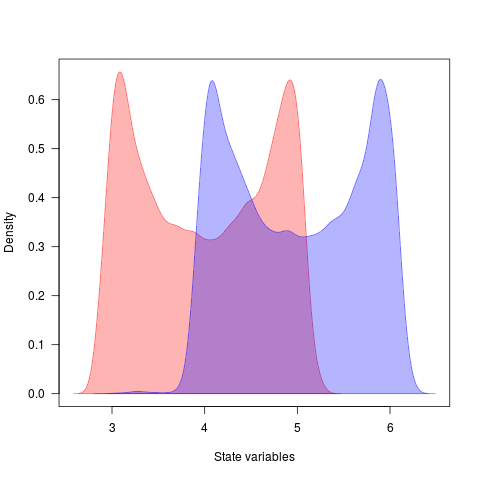or in two-dimensional space with

``````    TSDensity(ts.ex2, dim = 2)
``````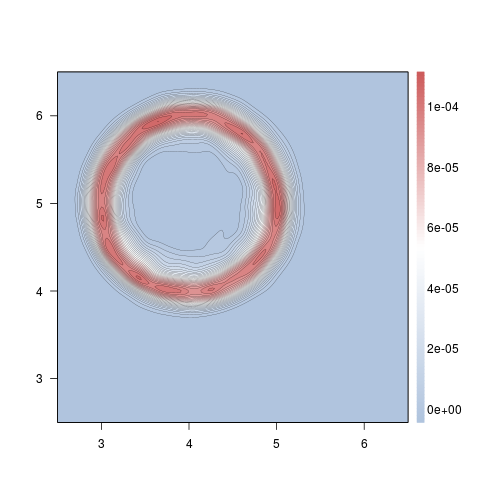### Step 3: Local quasi-potential calculations

To calculate the quasi-potential for a system of equations, we first need to define some initial conditions and parameter values.

``````    bounds.x = c(-0.5, 11.5)    # upper and lower limit of X
bounds.y = c(-0.5, 11.5)    # upper and lower limit of Y
step.number.x = 2000 #4000  # number of division between upper and lower limit
step.number.y = 2000 #4000  # number of division between upper and lower limit
xinit = 4.15611             # x value to start computing quasi-potential
yinit = 5.987774            # y value to start computing quasi-potential
``````

With these values defined, we can now compute the quasi-potential starting at the initial point. Note that we don't have to start at the equilibrium, but this ensures that we quickly find the lowest quasi-potential and work up from there.

``````    eq1.qp <- QPotential(x.rhs = parms.eqn.x, x.start = xinit, x.bound = bounds.x,
x.num.steps = step.number.x, y.rhs = parms.eqn.y, y.start = yinit,
y.bound = bounds.y, y.num.steps = step.number.y)
``````

The upwind ordered method will be chatty Set verboseC = FALSE to turn off completely Creating file name. File name created. Completed Memory Allocation equationx = -(y-5) + 0.2(x-4)(1-(x-4)2-(y-5)2) equationy = (x-4) + 0.2(y-5)(1-(x-4)2-(y-5)2) hx = 0.006003 hy = 0.006003 Finished initializing a bunch of matrices in param() function cputime = 45.9642 Finished Loading Parameters Finished ipoint() function Initial count = 4 current count = 2000 current count = 3000 current count = 5000 current count = 6000 current count = 7000 current count = 8000 current count = 7000 current count = 6000 current count = 5000 current count = 4000 current count = 3000 current count = 2000 current count = 3000 current count = 4000 current count = 5000 current count = 4000 current count = 3000 current count = 2000 current count = 3000 current count = 4000 current count = 5000 1756134 (2 916) is accepted, g=36.7663 Final count = 5983 Finished ordered_upwind() function cputime = 247.887 Saves only to R In datasave case 2 Successful. Exiting C code

### Step 4: Global quasi-potential calculation

In this example, the global quasi-potential is the same as the local quasi-potential computed above.

### Step 5: Global quasi-potential visualization

``````    QPContour(eq1.qp, dens = c(1000, 1000), x.bound = bounds.x, y.bound = bounds.y,
c.parm = 10, xlab=expression(italic(x)), ylab=expression(italic(y)))
``````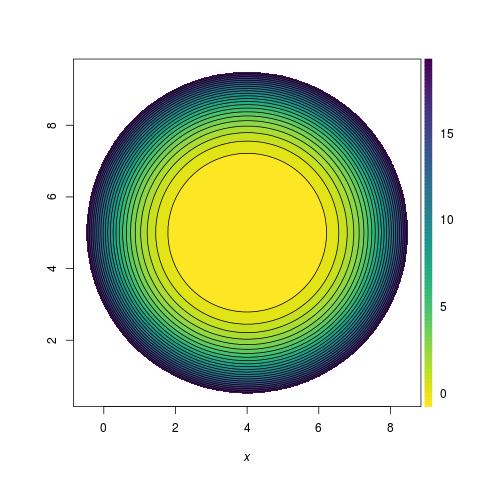In case you want to view the quasipotential in three dimensions, we can use the R package plot3D:

``````    require(plot3D); require(viridis)

#first, we have to subset the x and y axis because it is scaled from 0 to 1
frac.x <- c(0.2,0.5)
frac.y <- c(0.3,0.6)

#then we reduce the global quasi-potential matrix to contain only these values
ex1.global <- eq1.qp
global.sub <- ex1.global[round(dim(ex1.global)*frac.x):round(dim(ex1.global)*frac.x),
round(dim(ex1.global)*frac.y):round(dim(ex1.global)*frac.y)]

#regular data, can see the valley for the limit cycle
dens.sub <- c(200, 200) #pull only 200 rows and columns to speed up graphing
global.sub <- global.sub[round(seq(1,nrow(global.sub),length.out=dens.sub)) ,
round(seq(1,ncol(global.sub),length.out=dens.sub))]
global.sub[global.sub > 0.01] <- NA # limit the z axis to give the best shot
persp3D(z = global.sub, theta = 25, phi = 55, col = viridis(100, option = "C"), shade = 0.1,
colkey = list(side = 4, length = 0.85), contour = list(levels = c(0.01, 0.001, 0.0001, 0.00005 ) ),
zlim = c(-0.01, .011), zlab = intToUtf8(0x03A6))
#spend lots of time playing with theta, phi, zlim, contour levels, etc. to produce a decent graph
``````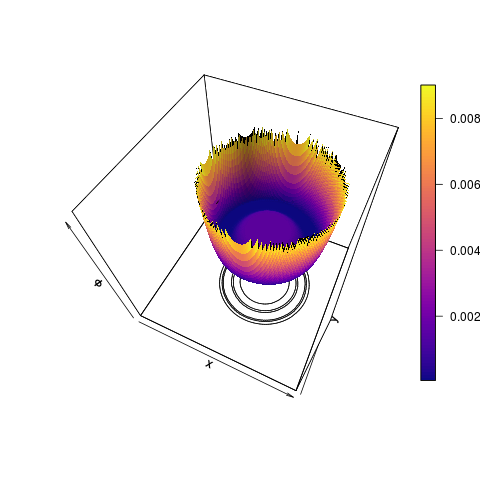To better see the valley for the limit cycle, we can ln-transform the quasi-potential and plot.

``````#natural log transformation
global.sub <- log(global.sub)
persp3D(z = global.sub, theta = 25, phi = 60, col = viridis(100, option = "C"), shade = 0.1,
colkey = list(side = 4, length = 0.85), contour = list(levels = seq(-10,-4,1) ), zlim = c(-15, -4),
zlab = paste("ln(",intToUtf8(0x03A6), ")", sep="")) # zlim = c(-10,-4)
``````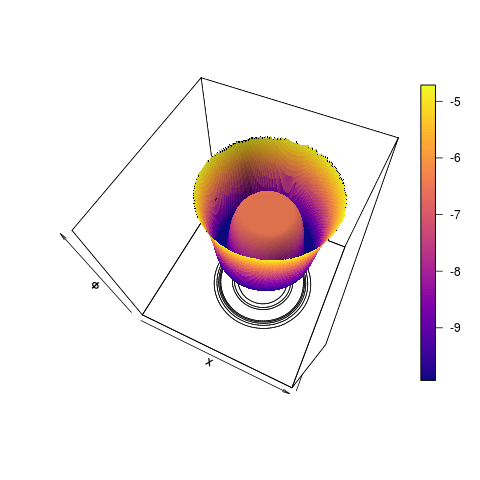### Step 6: Vector field decomposition

Taking the global quasi-potential, we can visualize the vector field, specifically the gradient and the remainder. First we calculate the vector field decomposition, which gives us the deterministic skeleton, the gradient vector field, and the remainder vector field for both state variables X and Y.

``````    VDAll <- VecDecomAll(surface = eq1.qp, x.rhs = parms.eqn.x,
y.rhs = parms.eqn.y, x.bound = bounds.x, y.bound = bounds.y)
``````

We can then plot the deterministic skeleton

``````    VecDecomPlot(x.field = VDAll[,,1], y.field = VDAll[,,2], dens = c(25, 25),
x.bound = bounds.x, y.bound = bounds.y, xlim = c(0, 11), ylim = c(0, 11),
arrow.type = "equal", tail.length = 0.25, head.length = 0.025)
``````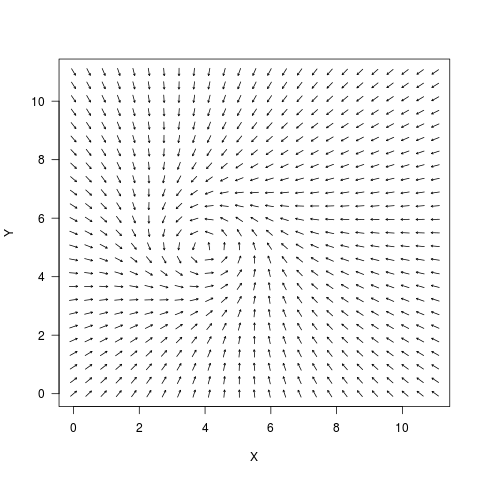followed by the gradient vector field

``````VecDecomPlot(x.field = VDAll[,,3], y.field = VDAll[,,4], dens = c(25, 25), x.bound = bounds.x,
y.bound = bounds.y, arrow.type = "proportional", tail.length = 0.25, head.length = 0.025)
``````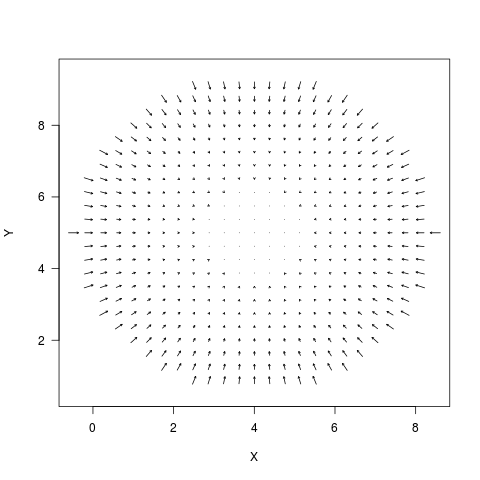and the remainder vector field, which is the “force that causes trajectories to circulate around level sets of the quasi-potential.” In this example, arrow.type = “proportional” and “equal” visually convey different aspects of the quasi-potential, where “equal” showcases the limit cycle and “proportional” showcases the variation in the gradient. First, the arrow.type = “proportional”:

``````VecDecomPlot(x.field = VDAll[,,5], y.field = VDAll[,,6], dens = c(25, 25), x.bound = bounds.x,
y.bound = bounds.y, arrow.type = "proportional", tail.length = 0.35, head.length = 0.025)
``````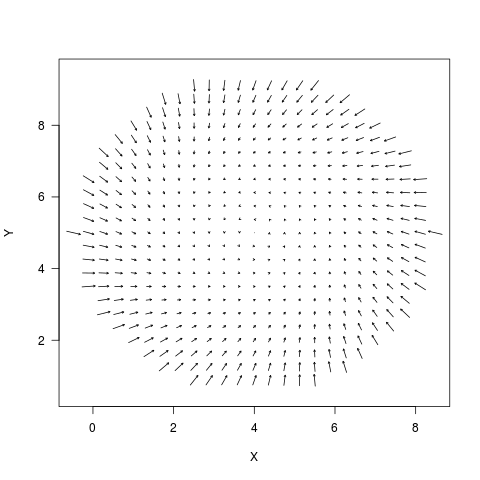Followed by the arrow.type = “equal”:

``````VecDecomPlot(x.field = VDAll[,,5], y.field = VDAll[,,6], dens = c(25, 25), x.bound = bounds.x,
y.bound = bounds.y, arrow.type = "equal", tail.length = 0.35, head.length = 0.025)
``````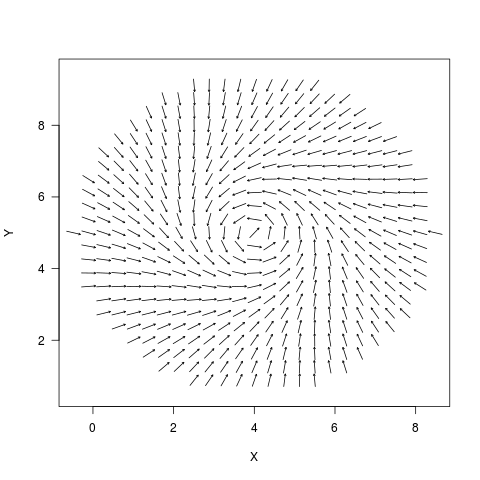The force arrows can be drawn equal size or can be drawn proportional to their value by setting arrow.type to “equal” or “proportional”.